# In the CS amplifier shown in the figure above, Rsig = 20 kΩ amplifier input resistance...

In the CS amplifier shown in the figure above, Rsig = 20 kΩ amplifier input resistance Rin = 80 kΩ, Cgs=2pF, Cgd= 0.5pF, gm=2mA/V, ro= 80 kΩ , RD = 5 kΩ, and RL = 10 kΩ. (π = 3.14)

a) Determine high frequency cut-off fH. (Use Miller Approximation. Use . as the decimal point. Answer in MHz; for 7.89MHz write 7.89 only.)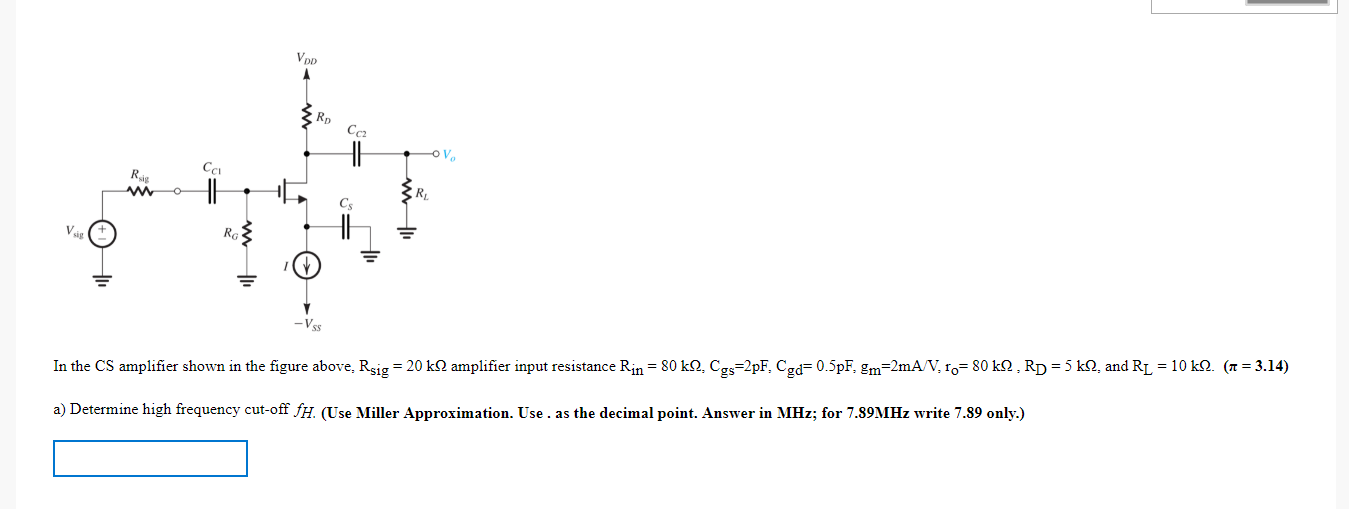VOD RD V RIS R CS V Ro In the CS amplifier shown in the figure above. Rsig = 20 kN amplifier input resistance Rin = 80 kN. Cgs=2pF. Cgd= 0.5pF. &m=2mA/V.1.=80k, Rp = 5 k1, and R1 = 10 k1. (1 = 3.14) a) Determine high frequency cut-off fH (Use Miller Approximation. Use . as the decimal point. Answer in MHz; for 7.89MHz write 7.89 only.)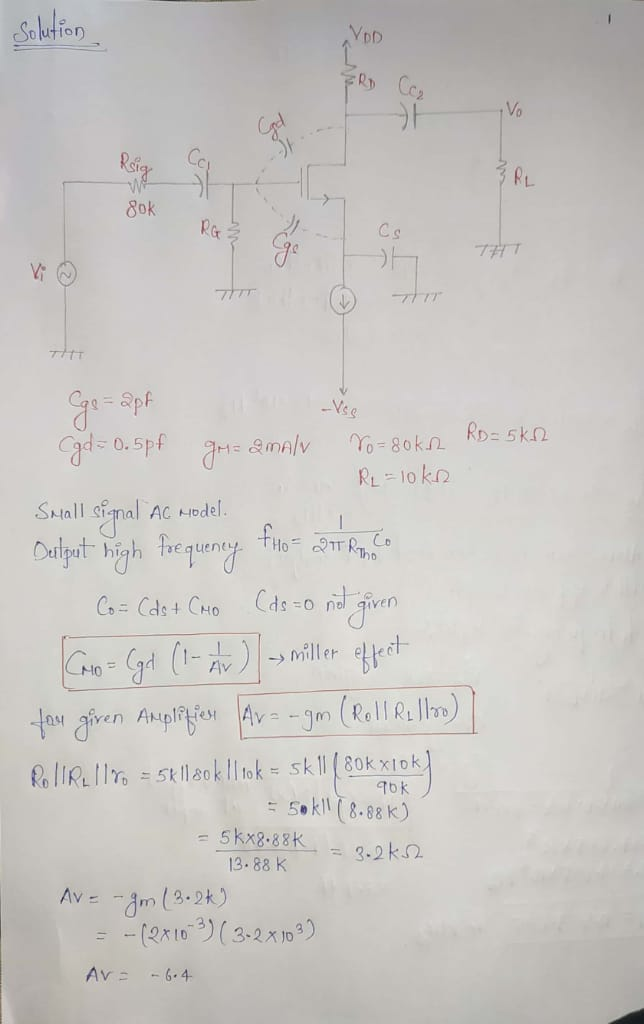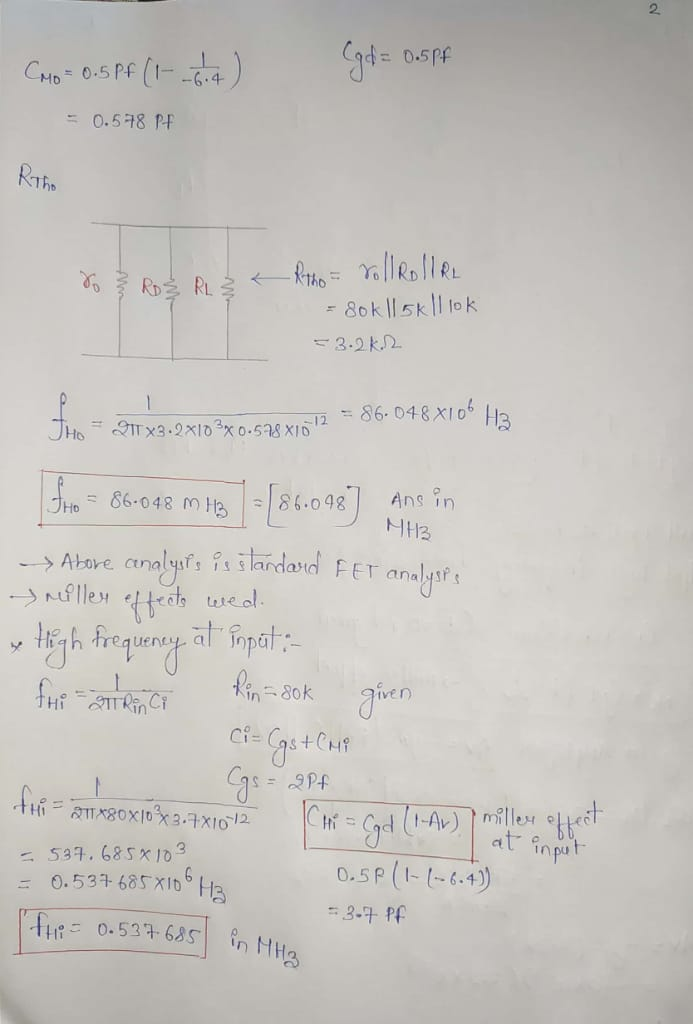plzzz don't give thumbs down.......plzzz comment if you have any problem i will try to solve your problem....plzz give thumbs up i am in need..

#### Earn Coin

Coins can be redeemed for fabulous gifts.

Similar Homework Help Questions
• ### Exercise 7.37: Design the bias circuit for the CS amplifier. Assume the MOSFET is specified to...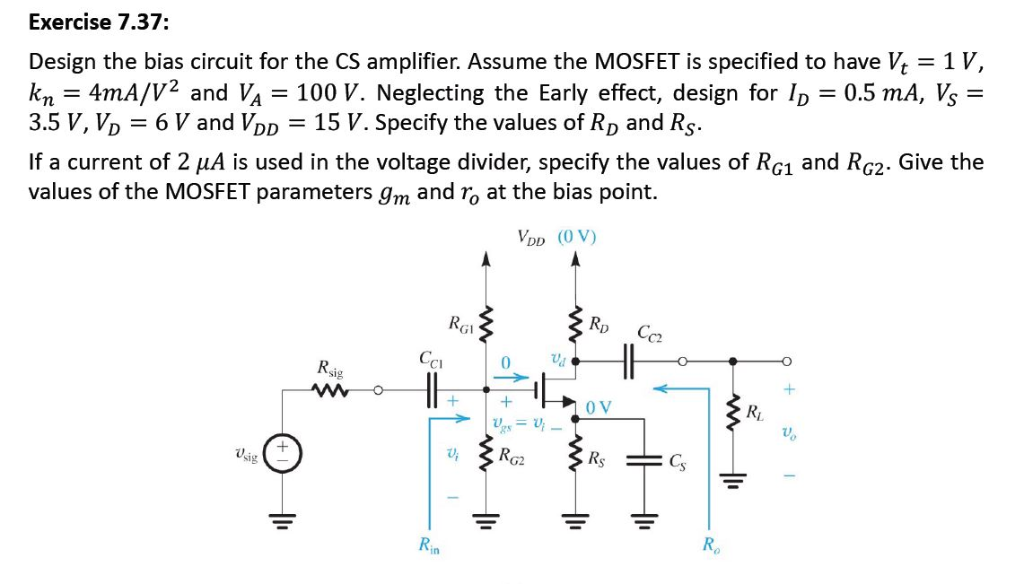Exercise 7.37: Design the bias circuit for the CS amplifier. Assume the MOSFET is specified to have Vt 1 V, kn = 4mA/V2 and V4 = 100 V. Neglecting the Early effect, design for ID-0.5mA, VS= 3.5 V, VD6 V and VDD 15 V. Specify the values of RD and Rs If a current of 2 μΑ is used in the voltage divider, specify the values of RG1 and RG2. Give the values of the MOSFET parameters gm and ro...

• ### The amplifier shown in Figure below has Rsig = R1 =1 k12, Rc= 1 k-2, RB...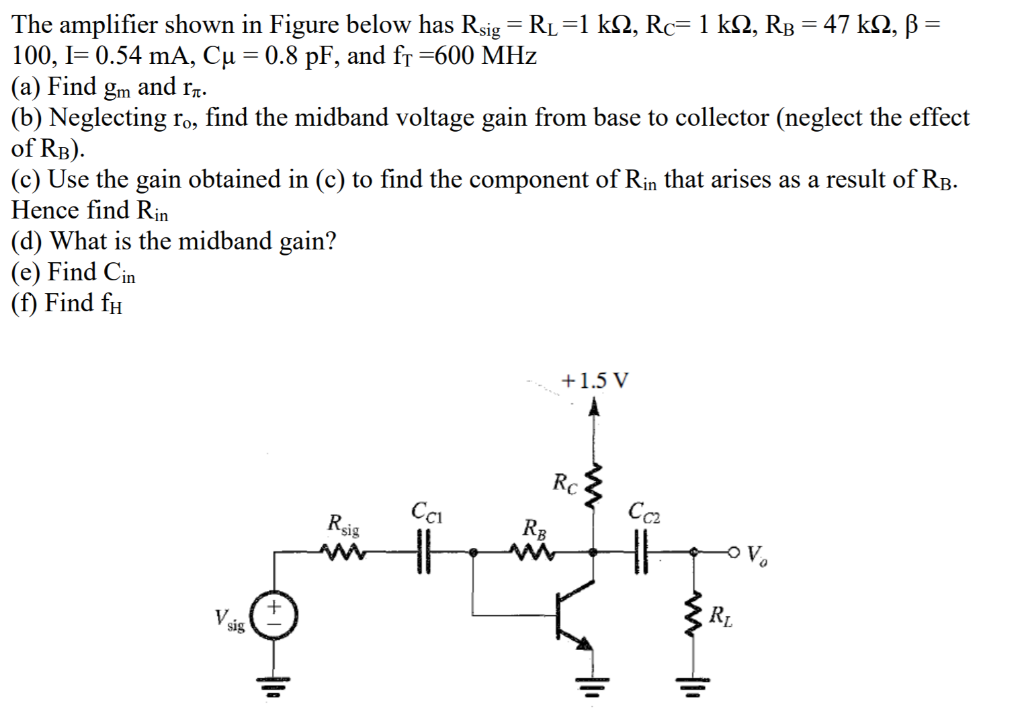The amplifier shown in Figure below has Rsig = R1 =1 k12, Rc= 1 k-2, RB = 47 k22, B = 100, 1=0.54 mA, Cu = 0.8 pF, and f1 =600 MHz (a) Find gm and r7. (b) Neglecting ro, find the midband voltage gain from base to collector (neglect the effect of RB). (c) Use the gain obtained in (c) to find the component of Rin that arises as a result of RB. Hence find Rin (d) What is...

• ### The NMOS transistor in the CS amplifier circuit of the figure is biased to have gn=4mA/V....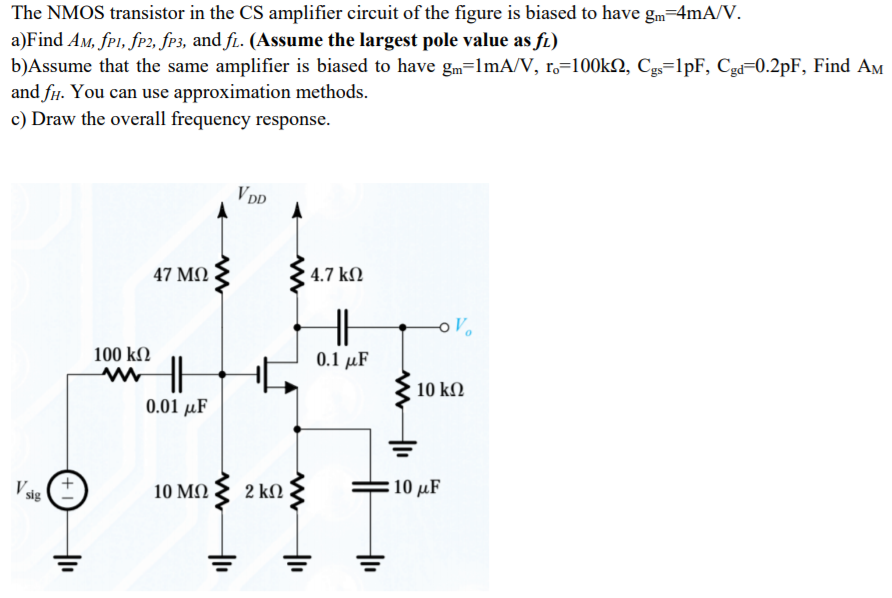The NMOS transistor in the CS amplifier circuit of the figure is biased to have gn=4mA/V. a)Find Am, fpi, fp2, fp3, and fl. (Assume the largest pole value as fl) b)Assume that the same amplifier is biased to have gm=lmA/V, 19=100k 2, Cgs=1pF, Cgd=0.2pF, Find Am and fu. You can use approximation methods. c) Draw the overall frequency response. VPD 47 ΜΩ 4.7 k12 w OV 100 k12 0.1 uF MH 10 k12 0.01 uF +1 10 ΜΩ 2 ΚΩ...

• ### Problem 3: Design Problem On Figure P3a, you have a Common Source (CS) n-channel MOSFET amplifier....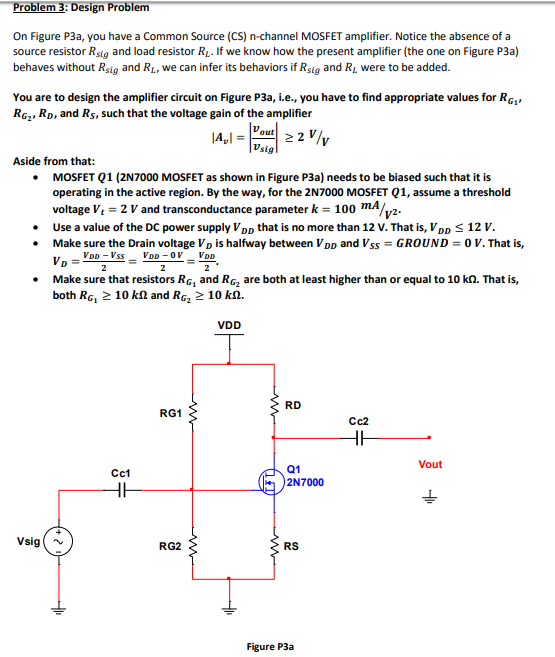Problem 3: Design Problem On Figure P3a, you have a Common Source (CS) n-channel MOSFET amplifier. Notice the absence of a source resistor Rsig and load resistor R. If we know how the present amplifier (the one on Figure P3a) behaves without Rsig and RL, we can infer its behaviors if Rsig and R were to be added. design the amplifier circuit on Figure P3a, i.e., you have to find appropriate values for RGj You are to RG,, RD, and...